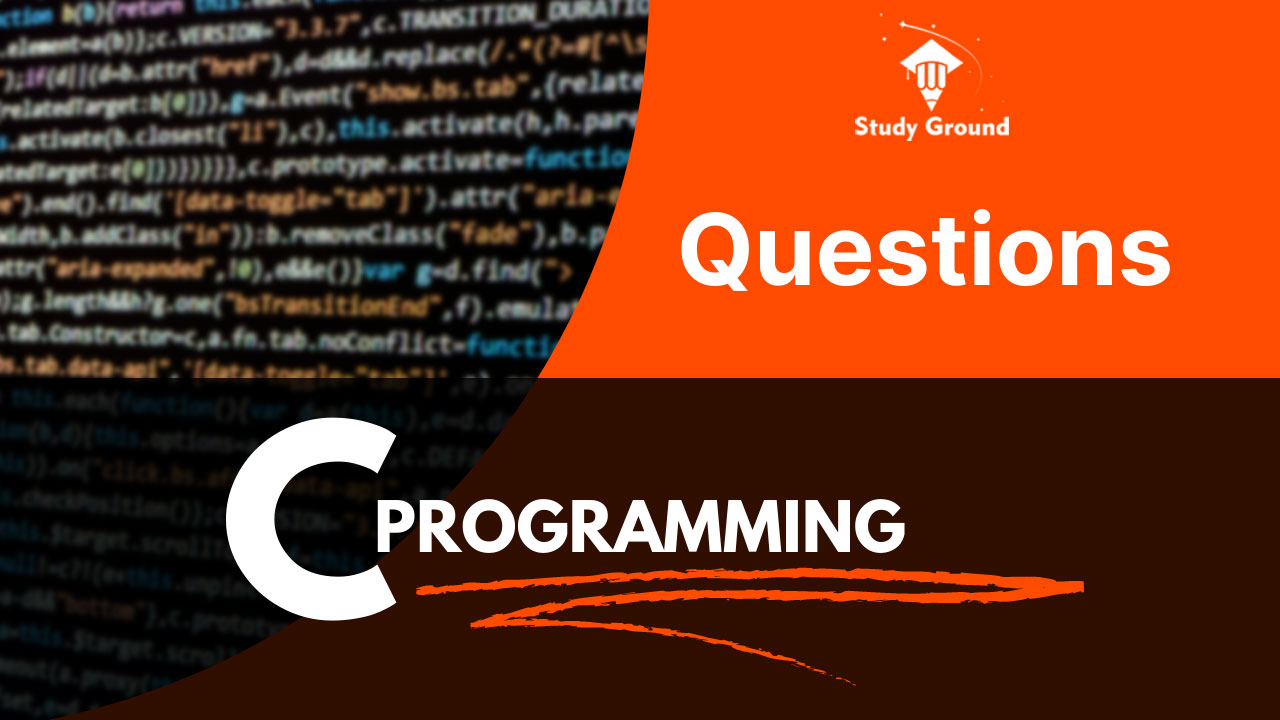# Basic Programming Questions on C Programming Language ( Part II )

The logic building is fundamental, to developing strong programming skills. It enables one to solve problems efficiently. Programming is essentially solving problems using code, and strong logic-building skills enable you to create efficient and effective solutions.

Here are some more questions which is the continuation of the previous questions. The Previous question link is here https://study-ground.com/article/59/basic-programming-questions-on-c-programming-language, we recommend you practice those questions first then try to solve the questions listed below.

Wish you good luck.

1. Write a C program to convert days into years,  weeks, and days
2. Write a C program to swap 2 numbers  with and without 3 rd variable
3. Write a C program to enter a radius of a circle and find its circumference and area.
4. What is the difference between a++ and  ++a? With an example
5. Write a C program to take input of 2 angles and find the third angle of a triangle.
6. Write a C program to take base the and height of  a triangle and find its area,
7. Write a C program to take input of marks of 5 subjects of a student calculate sum, average, percentage, and grade, and print the result in a tabular format.
8. Write a C program to convert one unit to another (for example litter to mill)
Tags: Programming Exercise Basic Programming Coding Practice Programming Skills C Programming Programming Challenges Coding Practice Beginner Programming Tasks Simple Coding Exercises Fundamental Programming Problems Basic Coding Drills Programming Puzzles For Beginners Entry-level Coding Assignments Programming Exercises For Beginners Coding Problems For Novices